# Graph Coloring: Working Through a Proof

#### (A new question of the week)

The Math Doctors have different levels of knowledge in various fields; I myself tend to focus on topics through calculus, which I know best, and leave the higher-level questions to others who are more recently familiar with them. But sometimes, both here and in my tutoring at a community college, I find that not being an expert can help me identify with a student, modeling for him how to make sense of something unfamiliar. That was true with this question about the chromatic number of a graph, a topic in graph theory (part of topology). It’s been years since I took topology, and I don’t recall whether I studied chromatic numbers even then. But the topic here is really “how to read a textbook proof”.

## The problem as presented

The question came to us in early December, with the title Graph Theory, under our Higher Math category:

Would be really obliged if anyone can help.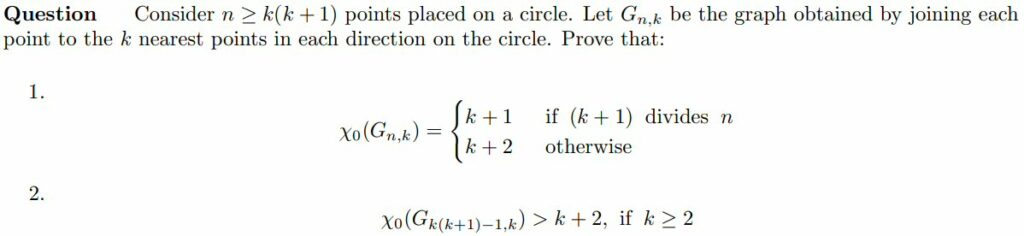The question sat unanswered for 24 hours, and I explained why when I answered:

Hi, Leonard.

The fact that none of us has answered yet suggests that we don’t have specific ideas about your problem, which is relatively specialized.

You would be much more likely to get help if you followed the request on our submission page, to “Show whatever work you have done, and tell us specifically where you need help.” Please show what you have tried, and also tell us what methods you have learned that might be relevant.

In particular, what is the definition of χ0 (I presume it is some sort of chromatic number, but I am not familiar with the subscript), and what theorems do you have that might be applied? Have you just been introduced to the concept, or have you learned special proof techniques? What is the context of the question more broadly?

Not being a graph theorist, but guessing that you might just need to apply the definition directly, my own inclination is to start by drawing a couple small examples of such graphs and think about what it would take to color them (assuming that is what the problem is about). That may give enough ideas.

Sometimes what looks like an immensely complicated problem is really fairly simple, and the best way to start is just to try something!

### Background: Graphs and chromatic numbers

Before moving on, I need to explain the terms in the question, all of which are easy to understand. First, a graph is a collection of points (called vertices or nodes) joined by lines (called edges or arcs):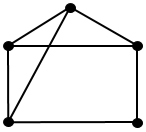This graph, which I’ll call A, has 5 vertices and 7 edges. In graph theory, we don’t care where or how large anything is, only how they are connected.

To color a graph, we assign each vertex a color; it isn’t really the colors we care about, only which are different and how many colors are needed. (In fact, often we think of colors as mere labels, like 1, 2, 3 or A, B, C as we will be doing below.) The full technical name for this is “proper vertex coloring”, “proper” meaning that no two adjacent vertices (joined by an edge) have the same color. Here is a process for coloring graph A using as few colors as possible: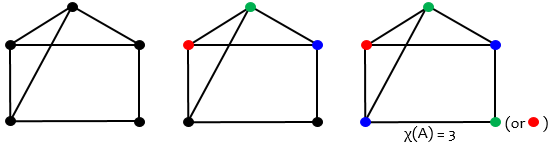I had to color the top three vertices differently (middle picture), because they are all connected to one another; this is called a clique (like a group of friends who all know each other). Then I had to color the lower left node blue, since it connects to both red and green; but it’s not connected to the existing blue. I chose to color the lower right node green, but I could have made it red. We couldn’t have used less than 3 colors, so we say the chromatic number of A, χ(A), is 3: the fewest colors possible. The symbol χ is the Greek letter chi, the first letter of “chromatic” in Greek. We say graph A is 3-colorable (k-colorable, in general).

What if we add another edge to make graph B?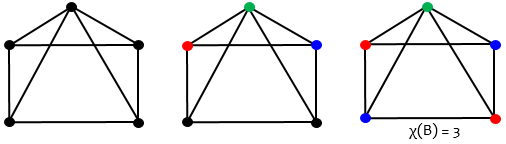This time I was forced to make the lower right node red, because it is joined to both of the other colors; but I still succeeded with 3 colors.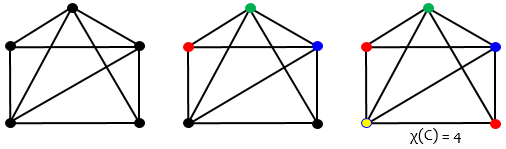This time the lower left node is connected to all three colors, so I was forced to use a fourth color, yellow. So the chromatic number of graph C is 4.

That’s just about all we’ll need to understand the problem!

## The real question: how to read a proof

My request drew out an unexpected response: both a corrected problem and a reversal of what we need to do:

Sorry, there was typo in the original question provided. Here is the book’s question: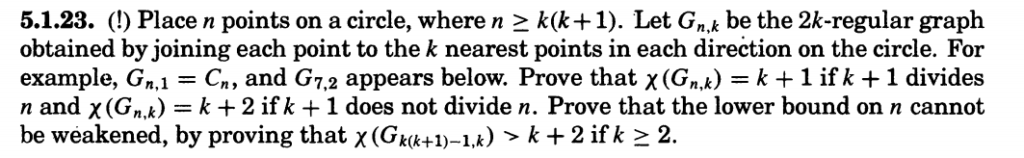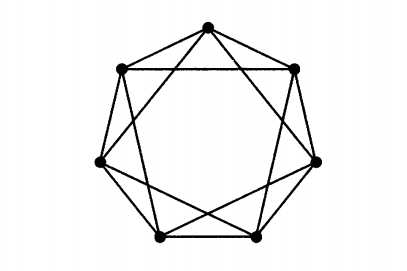Actually, I have the solution for this problem but I’m not able to understand it. I’m a beginner in graph theory and still trying to grasp concepts. Question is from book by Douglas B. West: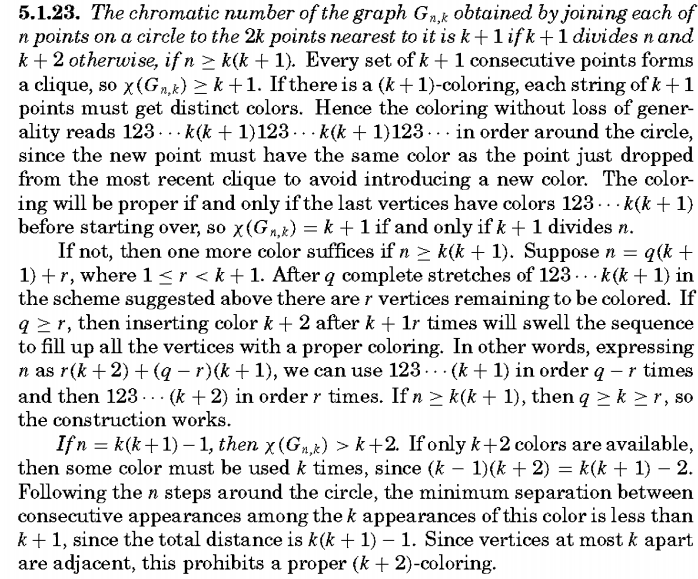Please provide some assistance in understanding it.

This helps a lot. Now we have the actual problem (of which the original version was a nicely simplified form), and an easier task (we hope): to understand the provided proof, which does indeed look intimidating at first glance. This puts it within my capability; now I can work alongside Leonard to figure it out. But I wasn’t quite ready to do that, as I wrote:

Thanks for all the additional information.

I need one more thing from you: Can you tell me at what point you have trouble understanding the solution?

If your difficulty starts at the very beginning, then what I would do to try to understand it is to take the example of G(7,2) and use that to make the problem and solution concrete. That is, first verify that it fits the description in the first couple sentences of the problem; then observe that k+1=3 does not divide n=7, so the claim is that the chromatic number is k+2=4. You might also try 4-coloring the graph, to confirm that it can be done; this will give you some sense of the issues that arise in doing so.

Then start working through the explanation of the solution, observing what each statement means applied to this graph. For example, observe that any 3 consecutive points form a clique (but this is not true of 4), and how that implies the graph can’t be colored with less than 3 colors. And so on.

This is what I am doing as I read through it myself!

When you respond with a brief statement of what you have done in reading the proof, and where you are stuck, we’ll be able to have a good discussion.

### Three small examples

Let’s start at the beginning, as I suggested, while we wait for Leonard to get back to us! Here is the setup for the theorem:

Place n points on a circle, where $$n \ge k(k+1)$$. Let $$G_{n,k}$$ be the 2k-regular graph obtained by joining each point to the k nearest points in each direction on the circle. For example, $$G_{n,1} = C_n$$, and $$G_{7,2}$$ appears below.

A 2k-regular graph is one in which each vertex has 2k edges coming from it; in this case, there are k on each side.

The graph $$C_n$$ is the cyclic graph (think of n people holding hands in a circle); here is our $$G_{7,1} = C_7$$, in which each vertex is joined to one on each side (making it 2-regular):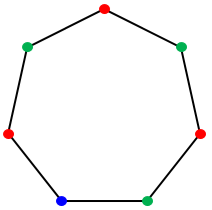For this example, $$n=7$$ and $$k=1$$, which satisfies the condition $$7 \ge 1(1+1)$$. Since 2 does not divide 7, the theorem claims that it should be 3-colorable ($$k+2=3$$), and we see that it is. (Can you see why it isn’t 2-colorable?)

Here is the graph $$G_{7,2}$$, with each vertex joined to 2 vertices on each side: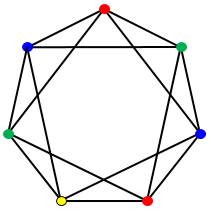For this example, $$n=7$$ and $$k=2$$, which satisfies the condition $$7 \ge 2(2+1)$$. Since 3 does not divide 7, the theorem claims that it should be 4-colorable ($$k+2=4$$). Good so far!

On the other hand, $$n=7$$ and $$k=3$$, does not satisfy the condition: $$7 < 3(3+1)$$, and in fact it is not 4-colorable as the theorem would have required if it applied: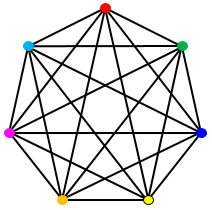Since every vertex is joined to every other, it takes 7 colors.

## The proof: first paragraph

Now, here is part 1 of the problem:

Prove that $$\chi(G_{n,k}) = k+1$$ if $$k+1$$ divides $$n$$ and $$\chi(G_{n,k}) = k+2$$ if $$k+1$$ does not divide $$n$$.

Because n in our examples is prime, both fit the second case, so that $$\chi(G_{n,k}) = k+2$$: $$\chi(G_{7,1}) = 1+2 = 3$$ and $$\chi(G_{7,2}) = 2+2=4$$. So the theorem is true for these examples. Did you think through how I colored them, and how those numbers arose?

Now we can examine the first paragraph of the provided solution, which I’ll break into sentences. For clarity, I’ll use $$G_{13,3}$$ as an illustration: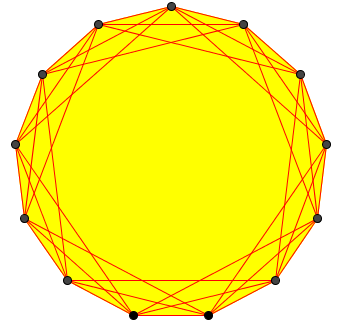Every set of $$k+1$$ consecutive points forms a clique, so $$\chi(G_{n,k})\ge k+1$$.

In the example, with k = 3, every four consecutive vertices form a clique, with each joined to each of the others; so we need at least that many colors: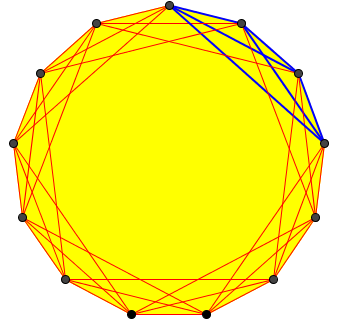If there is a $$(k+1)$$-coloring, each string of $$k+1$$ points must get distinct colors.

Here is the beginning of a coloring, using both colors and labels (A, B, C, D) for clarity:Hence the coloring, without loss of generality, reads $$123\cdots k(k+1)123\cdots k(k+1)123\cdots$$ in order around the circle, since the new point must have the same color as the point just dropped from the most recent clique to avoid introducing a new color.

Here I have continued the coloring all the way around; at the last place, I was forced to use a fifth color, E: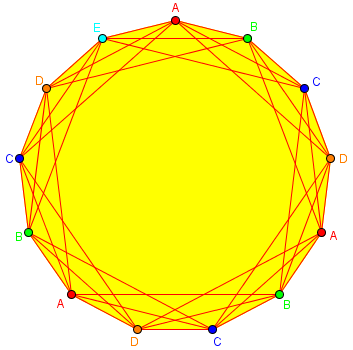The coloring will be proper if and only if the last vertices have colors $$123\cdots k(k+1)$$ before starting over, so $$\chi(G_{n,k})= k+1$$ if and only if $$k+1$$ divides $$n$$.

If the last set of 4 had fit exactly, the conflict wouldn’t have happened, so we would be done, as stated by the theorem. As it is, $$k+1$$ does not divide $$n$$, which was why we needed another color.

But there won’t always be just one left at the end; might we need more than one extra color?

### The second paragraph

This brings us to the point where we now find that Leonard is stuck:

Thanks for your reply, I understood part 1 of the question but I’m having a doubt how they have shown that “If n >= k(k+1) then q >= k >= r” and I have not understood how they are stating solution for the second part of the question when n = k(k+1) – 1.

Thanks

I didn’t have time just then to work through the whole proof, and was busy for much of the next 8 hours, so I sent an interim response just covering everything in the second paragraph except the sentence he is asking about:

Just so you know I’m not ignoring you, here is what I have written so far, and I’ll try to send more as soon as I can:

As I understand it, you are fine up to the last sentence of this paragraph: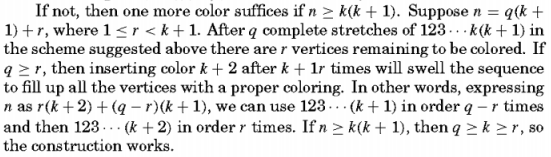Let’s take an example, working through the whole paragraph. Suppose k=3 and n=22, which is greater than 3(3+1). Then, dividing n by k+1, we find that q=5 and r=2, since 22=4(5)+2. We can color the vertices ABCD ABCD ABCD ABCD ABCD __, that is, 5 complete stretches of 4 colors, followed by r=2 vertices left to color. We can’t use any of colors A, B, C, D, but we can use E, putting it in 2 places: ABCD ABCD ABCD ABCDE ABCDE. This is a valid coloring, so we’ve shown that χ=5.

Now I’ll go look into that last sentence!

What I said here amounts to what we did above for $$G_{11,3}$$, leaving the last vertices uncolored. Here is that step for $$G_{22,3}$$, since I’m now using that example: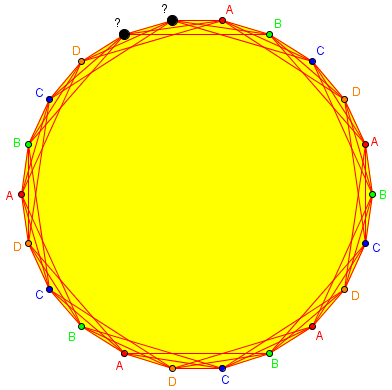If we kept things as they are, we’d need two more colors, as those last two can’t be the same. But if we spread the last couple vertices out, by skipping some earlier, then we can use one color, E, for both: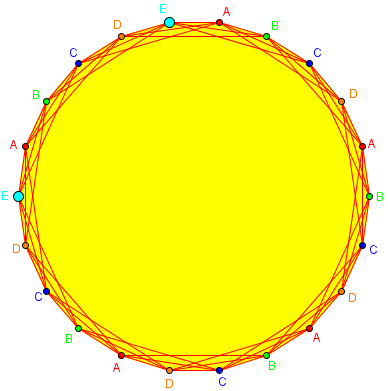The proof does the same things, but using variables to show that it can always be done.

### The last sentence

Once I had some time, I could write some more:

So that last sentence we’re trying to prove is:

If n ≥ k(k+1), then q ≥ k ≥ r, so the construction works.

In our example, this means that because 22 ≥ 3(3+1), then 5 ≥ 3 ≥ 1.

The remainder r is by definition less than the divisor k+1, so it is less than or equal to k. (My remainder, 1, had to be less than my divisor, 4, so it had to be no greater than my k, 3.) That explains the second inequality, k ≥ r.

How about q ≥ k? Look at the example first: Why did it have to be true that my quotient, 5, was no less than my k, 3 (which is 1 less than the divisor, 4)? Because my dividend, n=22, was big enough. But how big is big enough? For the quotient to be at least 3, the dividend has to be at least 3 times the divisor, 4, namely 12 (which is k(k+1)). If I’d used n=12, the quotient would have been 3, which is big enough.

But there’s a little twist in proving this in general.

We defined q, the quotient, by the Division Algorithm, n = q(k + 1) + r; so the assumption n ≥ k(k+1) implies that q(k + 1) + r ≥ k(k+1), that is, q(k + 1) – k(k+1) ≥ -r, or (q – k)(k+1) ≥ -r. So q – k ≥ -r/(k + 1). That doesn’t quite get us to the goal yet!

But since r < k + 1, we know that r/(k + 1) < 1; and since q – k is an integer, q – k ≥ -r/(k + 1) > -1 implies that q – k ≥ 0 because there is no integer between -1 and 0! Therefore q ≥ k. Q.E.D.

I’ll look at the second part of the problem next!

Considering our example with $$n=22$$ and $$k=3$$, we divided by 4, leaving quotient $$q=5$$ and remainder $$r=2$$. Our $$-r/(k + 1)$$ is $$-2/(3+1)=-\frac{1}{2}$$. The next larger integer is 0, so we can conclude that $$q-k\ge 0$$ as we need. The fact that the fraction was negative didn’t allow q to be less than k.

## The third paragraph

Here is the second part of the theorem:

Prove that the lower bound on n cannot be weakened, by proving that $$\chi(G_{k(k+1)-1,k}) > k+2$$ if $$k\ge 2$$.

That is, we couldn’t replace the condition $$n \ge k(k+1)$$ in the first part with any smaller number, because it is possible to need more than $$k+2$$ colors if we decrease that bound even by 1.

Another half hour’s work, and I had that worked out:

Here’s their proof for the second part: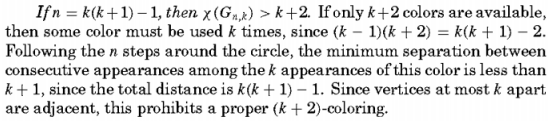We’re trying to show that if n is less than required for the first part, then it will no longer be true that we never need more than k+2 colors.

So we try to color the graph with k+2 colors, and see what goes wrong. This time, suppose k=3 and n=11, which is 1 less than 3(3+1), and we try coloring with 5 colors. After using each color twice, we’ve colored only 10 vertices, so we have to use some color at least 3 times. The first 4 colors can be placed like A B C D A B C D, and we need to fit in 3 E’s somewhere in between. But there are only 8 places to put them, and they have to be 4 apart (since any 4 consecutive vertices form a clique): E _ _ _ E _ _ _ E _ _ _, and that would require 12 vertices (k(k+1) = 3*4). Something has to be squeezed into two vertices that are adjacent.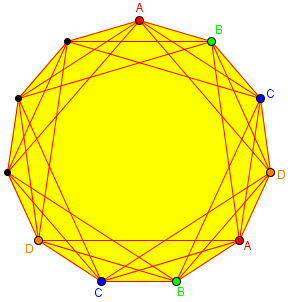We could put E anywhere, but the next has to be 4 places further, and then there is no room for the third E: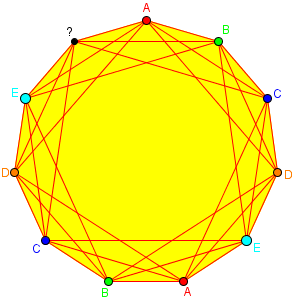The next day, we were done:

Thanks doctor for you reply, it really helped me a lot. 🙂

Thanks

Leonard

### 1 thought on “Graph Coloring: Working Through a Proof”

This site uses Akismet to reduce spam. Learn how your comment data is processed.